# Some circular summation formulas for theta functions

## Abstract

In this paper, we obtain some circular summation formulas of theta functions using the theory of elliptic functions and show some interesting identities of theta functions and applications.

MSC:11F27, 33E05, 11F20.

## 1 Introduction

Throughout this paper we take $q={e}^{i\pi \tau }$, where $\mathrm{\Im }\left(\tau \right)>0$. The classical Jacobi theta functions ${\theta }_{i}\left(z|\tau \right)$, $i=1,2,3,4$, are defined as follows:(1.1)(1.2)(1.3)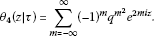(1.4)

Recently, Chan, Liu and Ng  proved Ramanujan’s circular summation formulas and derived identities similar to Ramanujan’s summation formula and connected these identities to Jacobi’s elliptic functions.

Subsequently, Zeng  gave a generalized circular summation of the theta function ${\theta }_{3}\left(z|\tau \right)$ as follows:(1.5)

where

${\mathcal{C}}_{33}\left(a,b;y,\tau \right)=kn\underset{{m}_{1},\dots ,{m}_{a},{n}_{1},\dots ,{n}_{b}=-\mathrm{\infty }}{\overset{\mathrm{\infty }}{\sum _{{m}_{1}+\cdots +{m}_{a}+{n}_{1}+\cdots +{n}_{b}=0}}}{q}^{{m}_{1}^{2}+\cdots +{m}_{a}^{2}+{n}_{1}^{2}+\cdots +{n}_{b}^{2}}{e}^{2k\left({m}_{1}+\cdots +{m}_{a}\right)iy}.$

A special case of formula (1.5) yields the following result (see [, Theorem 3.1]):

$\sum _{s=0}^{kn-1}{\theta }_{3}^{k}\left(\frac{z}{kn}+\frac{\pi s}{kn}|\frac{\tau }{k{n}^{2}}\right)={\mathcal{C}}_{33}\left(k,0;0,\frac{\tau }{k{n}^{2}}\right){\theta }_{3}\left(z|\tau \right),$
(1.6)

where

${\mathcal{C}}_{33}\left(k,0;0,\tau \right)=kn\underset{{m}_{1},\dots ,{m}_{k}=-\mathrm{\infty }}{\overset{\mathrm{\infty }}{\sum _{{m}_{1}+\cdots +{m}_{k}=0}}}{q}^{{m}_{1}^{2}+\cdots +{m}_{k}^{2}}.$
(1.7)

Upon a, b, n and k are any positive integer with $k=a+b$.

More recently, Liu further obtained the general formulas for theta functions (see ), but from one main result, Theorem 1 of Liu, we do not deduce our results in the present paper. Many people research the circular summation formulas of theta functions and find more interesting formulas (see, for details, ).

In the present paper, we obtain analogues and uniform formulas for theta functions ${\theta }_{1}\left(z|\tau \right)$, $a{\theta }_{2}\left(z|\tau \right)$, ${\theta }_{3}\left(z|\tau \right)$ and ${\theta }_{4}\left(z|\tau \right)$. We now state our result as follows.

Theorem 1 For any positive integer k, n, a and b with $k=a+b$, $\alpha =1,2$, $\beta =3,4$.

• For a, b even, we have(1.8)
• For a even, n and b odd, we have(1.9)(1.10)

where

$\begin{array}{rcl}{\mathcal{C}}_{\alpha \beta }\left(a,b;y,\tau \right)& =& kn{i}^{a\alpha }{q}^{\frac{a}{4}}{e}^{abiy}\underset{2\left({m}_{1}+\cdots +{m}_{a}+{n}_{1}+\cdots +{n}_{b}\right)+a=0}{\overset{\mathrm{\infty }}{\sum _{{m}_{1},\dots ,{m}_{a},{n}_{1},\dots ,{n}_{b}=-\mathrm{\infty }}}}{\left(-1\right)}^{\alpha \left({m}_{1}+\cdots +{m}_{a}\right)+\left(\beta +1\right)\left({n}_{1}+\cdots +{n}_{b}\right)}\\ ×{q}^{{m}_{1}^{2}+\cdots +{m}_{a}^{2}+{n}_{1}^{2}+\cdots +{n}_{b}^{2}+{m}_{1}+\cdots +{m}_{a}}{e}^{\left(2k\left({m}_{1}+\cdots +{m}_{a}\right)+{a}^{2}\right)iy}.\end{array}$
(1.11)

## 2 Proof of Theorem 1

From Jacobi’s theta functions (1.1)-(1.4), we have the following properties respectively:(2.1)(2.2)(2.3)(2.4)

From (2.1)-(2.4), by using the induction, we easily obtain(2.5)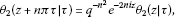(2.6)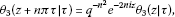(2.7)(2.8)

Let

$f\left(z\right)=\sum _{s=0}^{kn-1}{\theta }_{\alpha }^{a}\left(\frac{z}{kn}+\frac{y}{a}+\frac{\pi s}{kn}|\frac{\tau }{k{n}^{2}}\right){\theta }_{\beta }^{b}\left(\frac{z}{kn}-\frac{y}{b}+\frac{\pi s}{kn}|\frac{\tau }{k{n}^{2}}\right).$
(2.9)

Case 1. When $\alpha =1$, $\beta =3$.

The function $f\left(z\right)$ becomes of the following form:

$f\left(z\right)=\sum _{s=0}^{kn-1}{\theta }_{1}^{a}\left(\frac{z}{kn}+\frac{y}{a}+\frac{\pi s}{kn}|\frac{\tau }{k{n}^{2}}\right){\theta }_{3}^{b}\left(\frac{z}{kn}-\frac{y}{b}+\frac{\pi s}{kn}|\frac{\tau }{k{n}^{2}}\right).$
(2.10)

From (2.10) we easily obtain(2.11)(2.12)

Comparing (2.11) and (2.12), when a is even, we get

$f\left(z+\pi \right)=f\left(z\right).$
(2.13)

By (2.5) and (2.7), and noting that $a+b=k$, we obtain

$\begin{array}{rcl}f\left(z+\pi \tau \right)& =& \sum _{s=0}^{kn-1}{\theta }_{1}^{a}\left(\frac{z}{kn}+\frac{y}{a}+\frac{\pi s}{kn}+n\pi \frac{\tau }{k{n}^{2}}|\frac{\tau }{k{n}^{2}}\right){\theta }_{3}^{b}\left(\frac{z}{kn}-\frac{y}{b}+\frac{\pi s}{kn}+n\pi \frac{\tau }{k{n}^{2}}|\frac{\tau }{k{n}^{2}}\right)\\ =& {\left(-1\right)}^{na}{q}^{-1}{e}^{-2iz}\sum _{s=0}^{kn-1}{\theta }_{1}^{a}\left(\frac{z}{kn}+\frac{y}{a}+\frac{\pi s}{kn}|\frac{\tau }{k{n}^{2}}\right){\theta }_{3}^{b}\left(\frac{z}{kn}-\frac{y}{b}+\frac{\pi s}{kn}|\frac{\tau }{k{n}^{2}}\right).\end{array}$
(2.14)

Obviously, when a is even, we have

$f\left(z+\pi \tau \right)={q}^{-1}{e}^{-2iz}f\left(z\right).$
(2.15)

We construct the function $\frac{f\left(z\right)}{{\theta }_{3}\left(z|\tau \right)}$. By (2.13) and (2.15), we find that the function $\frac{f\left(z\right)}{{\theta }_{3}\left(z|\tau \right)}$ is an elliptic function with double periods π and πτ and only has a simple pole at $z=\frac{\pi }{2}+\frac{\pi \tau }{2}$ in the period parallelogram. Hence the function $\frac{f\left(z\right)}{{\theta }_{3}\left(z|\tau \right)}$ is a constant, say, this constant is denoted by ${C}_{13}\left(a,b;y,\tau \right)$, i.e.,

$\frac{f\left(z\right)}{{\theta }_{3}\left(z|\tau \right)}={C}_{13}\left(a,b;y,\tau \right),$

we have

$f\left(z\right)={C}_{13}\left(a,b;y,\tau \right){\theta }_{3}\left(z|\tau \right).$
(2.16)

By (1.3), (2.10) and (2.16), we have(2.17)

By (1.1) and (1.3), we obtain(2.18)

By equating the constant term of both sides of (2.18), we obtain(2.19)

Clearly,

${C}_{13}\left(a,b;y,\tau \right)={\mathcal{C}}_{13}\left(a,b;\frac{y}{ab},\frac{\tau }{k{n}^{2}}\right),$
(2.20)

where(2.21)

In the same manner as in Case 1, we can obtain Case 2 below.

Case 2. When $\alpha =2$, $\beta =3$.

The function $f\left(z\right)$ becomes of the following form:

$f\left(z\right)=\sum _{s=0}^{kn-1}{\theta }_{2}^{a}\left(\frac{z}{kn}+\frac{y}{a}+\frac{\pi s}{kn}|\frac{\tau }{k{n}^{2}}\right){\theta }_{3}^{b}\left(\frac{z}{kn}-\frac{y}{b}+\frac{\pi s}{kn}|\frac{\tau }{k{n}^{2}}\right).$
(2.22)

From (2.22) we easily obtain(2.23)(2.24)

Comparing (2.23) and (2.24), when a is even, we get

$f\left(z+\pi \right)=f\left(z\right).$
(2.25)

By (2.6) and (2.7), and noting that $a+b=k$, we obtain

$\begin{array}{rcl}f\left(z+\pi \tau \right)& =& \sum _{s=0}^{kn-1}{\theta }_{2}^{a}\left(\frac{z}{kn}+\frac{y}{a}+\frac{\pi s}{kn}+n\pi \frac{\tau }{k{n}^{2}}|\frac{\tau }{k{n}^{2}}\right){\theta }_{3}^{b}\left(\frac{z}{kn}-\frac{y}{b}+\frac{\pi s}{kn}+n\pi \frac{\tau }{k{n}^{2}}|\frac{\tau }{k{n}^{2}}\right)\\ =& {q}^{-1}{e}^{-2iz}\sum _{s=0}^{kn-1}{\theta }_{2}^{a}\left(\frac{z}{kn}+\frac{y}{a}+\frac{\pi s}{kn}|\frac{\tau }{k{n}^{2}}\right){\theta }_{3}^{b}\left(\frac{z}{kn}-\frac{y}{b}+\frac{\pi s}{kn}|\frac{\tau }{k{n}^{2}}\right).\end{array}$
(2.26)

Obviously, we have

$f\left(z+\pi \tau \right)={q}^{-1}{e}^{-2iz}f\left(z\right).$
(2.27)

We construct the function $\frac{f\left(z\right)}{{\theta }_{3}\left(z|\tau \right)}$. By (2.25) and (2.27), we find that the function $\frac{f\left(z\right)}{{\theta }_{3}\left(z|\tau \right)}$ is an elliptic function with double periods π and πτ and only has a simple pole at $z=\frac{\pi }{2}+\frac{\pi \tau }{2}$ in the period parallelogram. Hence the function $\frac{f\left(z\right)}{{\theta }_{3}\left(z|\tau \right)}$ is a constant, say, this constant is denoted by ${C}_{23}\left(a,b;y,\tau \right)$, i.e.,

$\frac{f\left(z\right)}{{\theta }_{3}\left(z|\tau \right)}={C}_{23}\left(a,b;y,\tau \right),$

we have

$f\left(z\right)={C}_{23}\left(a,b;y,\tau \right){\theta }_{3}\left(z|\tau \right).$
(2.28)

By (1.3), (2.22) and (2.28), we have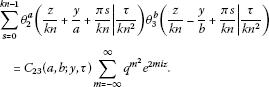(2.29)

By (1.2) and (1.3), we obtain(2.30)

By equating the constant term of both sides of (2.30), we obtain

$\begin{array}{rcl}{C}_{23}\left(a,b;y,\tau \right)& =& kn{q}^{\frac{a}{4k{n}^{2}}}{e}^{iy}\underset{2\left({m}_{1}+\cdots +{m}_{a}+{n}_{1}+\cdots +{n}_{b}\right)+a=0}{\overset{\mathrm{\infty }}{\sum _{{m}_{1},\dots ,{m}_{a},{n}_{1},\dots ,{n}_{b}=-\mathrm{\infty }}}}{q}^{\frac{{m}_{1}^{2}+\cdots +{m}_{a}^{2}+{n}_{1}^{2}+\cdots +{n}_{b}^{2}+{m}_{1}+\cdots +{m}_{a}}{k{n}^{2}}}\\ ×{e}^{\frac{2iy}{ab}\left[k\left({m}_{1}+\cdots +{m}_{a}\right)+\frac{{a}^{2}}{2}\right]}.\end{array}$
(2.31)

Clearly,

${C}_{23}\left(a,b;y,\tau \right)={\mathcal{C}}_{23}\left(a,b;\frac{y}{ab},\frac{\tau }{k{n}^{2}}\right),$
(2.32)

where

$\begin{array}{rcl}{\mathcal{C}}_{23}\left(a,b;y,\tau \right)& =& kn{q}^{\frac{a}{4}}{e}^{abiy}\sum _{\begin{array}{c}{m}_{1},\dots ,{m}_{a},{n}_{1},\dots ,{n}_{b}=-\mathrm{\infty }\\ 2\left({m}_{1}+\cdots +{m}_{a}+{n}_{1}+\cdots +{n}_{b}\right)+a=0\end{array}}^{\mathrm{\infty }}{q}^{{m}_{1}^{2}+\cdots +{m}_{a}^{2}+{n}_{1}^{2}+\cdots +{n}_{b}^{2}+{m}_{1}+\cdots +{m}_{a}}\\ ×{e}^{\left(2k\left({m}_{1}+\cdots +{m}_{a}\right)+{a}^{2}\right)iy}.\end{array}$
(2.33)

Case 3. When $\alpha =1$, $\beta =4$.

The function $f\left(z\right)$ becomes of the following form:

$f\left(z\right)=\sum _{s=0}^{kn-1}{\theta }_{1}^{a}\left(\frac{z}{kn}+\frac{y}{a}+\frac{\pi s}{kn}|\frac{\tau }{k{n}^{2}}\right){\theta }_{4}^{b}\left(\frac{z}{kn}-\frac{y}{b}+\frac{\pi s}{kn}|\frac{\tau }{k{n}^{2}}\right).$
(2.34)

From (2.34) we easily obtain(2.35)(2.36)

Comparing (2.35) and (2.36), when a is even, we have

$f\left(z+\pi \right)=f\left(z\right).$
(2.37)

By (2.5) and (2.8), and noting that $a+b=k$, we obtain

$\begin{array}{rcl}f\left(z+\pi \tau \right)& =& \sum _{s=0}^{kn-1}{\theta }_{1}^{a}\left(\frac{z}{kn}+\frac{y}{a}+\frac{\pi s}{kn}+n\pi \frac{\tau }{k{n}^{2}}|\frac{\tau }{k{n}^{2}}\right){\theta }_{4}^{b}\left(\frac{z}{kn}-\frac{y}{b}+\frac{\pi s}{kn}+n\pi \frac{\tau }{k{n}^{2}}|\frac{\tau }{k{n}^{2}}\right)\\ =& {\left(-1\right)}^{kn}{q}^{-1}{e}^{-2iz}\sum _{s=0}^{kn-1}{\theta }_{1}^{a}\left(\frac{z}{kn}+\frac{y}{a}+\frac{\pi s}{kn}|\frac{\tau }{k{n}^{2}}\right){\theta }_{4}^{b}\left(\frac{z}{kn}-\frac{y}{b}+\frac{\pi s}{kn}|\frac{\tau }{k{n}^{2}}\right).\end{array}$
(2.38)
• When a and b are even, then kn is also even, we have

$f\left(z+\pi \tau \right)={q}^{-1}{e}^{-2iz}f\left(z\right).$
(2.39)

We construct the function $\frac{f\left(z\right)}{{\theta }_{3}\left(z|\tau \right)}$, by (2.37) and (2.39), we find that the function $\frac{f\left(z\right)}{{\theta }_{3}\left(z|\tau \right)}$ is an elliptic function with double periods π and πτ and only has a simple pole at $z=\frac{\pi }{2}+\frac{\pi \tau }{2}$ in the period parallelogram. Hence the function $\frac{f\left(z\right)}{{\theta }_{3}\left(z|\tau \right)}$ is a constant, say, this constant is denoted by ${C}_{14}\left(a,b;y,\tau \right)$, i.e.,

$\frac{f\left(z\right)}{{\theta }_{3}\left(z|\tau \right)}={C}_{14}\left(a,b;y,\tau \right),$

we have

$f\left(z\right)={C}_{14}\left(a,b;y,\tau \right){\theta }_{3}\left(z|\tau \right).$
(2.40)

By (1.3), (2.34) and (2.40), we have(2.41)

By (1.1) and (1.4), we obtain(2.42)

By equating the constant term of both sides of (2.42), we obtain

$\begin{array}{rcl}{C}_{14}\left(a,b;y,\tau \right)& =& kn{i}^{a}{q}^{\frac{a}{4k{n}^{2}}}{e}^{iy}\underset{2\left({m}_{1}+\cdots +{m}_{a}+{n}_{1}+\cdots +{n}_{b}\right)+a=0}{\overset{\mathrm{\infty }}{\sum _{{m}_{1},\dots ,{m}_{a},{n}_{1},\dots ,{n}_{b}=-\mathrm{\infty }}}}{\left(-1\right)}^{{m}_{1}+\cdots +{n}_{b}}\\ ×{q}^{\frac{{m}_{1}^{2}+\cdots +{m}_{a}^{2}+{n}_{1}^{2}+\cdots +{n}_{b}^{2}+{m}_{1}+\cdots +{m}_{a}}{k{n}^{2}}}{e}^{\frac{2iy}{ab}\left[k\left({m}_{1}+\cdots +{m}_{a}\right)+\frac{{a}^{2}}{2}\right]}.\end{array}$
(2.43)
• When a is even, n and b are odd, then kn is also odd, we have

$f\left(z+\pi \tau \right)=-{q}^{-1}{e}^{-2iz}f\left(z\right).$
(2.44)

We construct the function $\frac{f\left(z\right)}{{\theta }_{4}\left(z|\tau \right)}$. By (2.37) and (2.44), we find that the function $\frac{f\left(z\right)}{{\theta }_{4}\left(z|\tau \right)}$ is an elliptic function with double periods π and πτ and only has a simple pole at $z=\frac{\pi }{2}+\frac{\pi \tau }{2}$ in the period parallelogram. Hence the function $\frac{f\left(z\right)}{{\theta }_{4}\left(z|\tau \right)}$ is a constant, say, this constant is denoted by ${C}_{14}\left(a,b;y,\tau \right)$, i.e.,

$\frac{f\left(z\right)}{{\theta }_{4}\left(z|\tau \right)}={C}_{14}\left(a,b;y,\tau \right),$

we have

$f\left(z\right)={C}_{14}\left(a,b;y,\tau \right){\theta }_{4}\left(z|\tau \right).$
(2.45)

By (1.4), (2.34) and (2.45), we have(2.46)

By (1.1) and (1.4), we obtain(2.47)

By equating the constant term of both sides of (2.47), we obtain

$\begin{array}{rcl}{C}_{14}\left(a,b;y,\tau \right)& =& kn{i}^{a}{q}^{\frac{a}{4k{n}^{2}}}{e}^{iy}\sum _{\begin{array}{c}{m}_{1},\dots ,{m}_{a},{n}_{1},\dots ,{n}_{b}=-\mathrm{\infty }\\ 2\left({m}_{1}+\cdots +{m}_{a}+{n}_{1}+\cdots +{n}_{b}\right)+a=0\end{array}}^{\mathrm{\infty }}{\left(-1\right)}^{{m}_{1}+\cdots +{n}_{b}}\\ ×{q}^{\frac{{m}_{1}^{2}+\cdots +{m}_{a}^{2}+{n}_{1}^{2}+\cdots +{n}_{b}^{2}+{m}_{1}+\cdots +{m}_{a}}{k{n}^{2}}}{e}^{\frac{2iy}{ab}\left[k\left({m}_{1}+\cdots +{m}_{a}\right)+\frac{{a}^{2}}{2}\right]}.\end{array}$
(2.48)

Clearly, in (2.43) and (2.48), we have

${C}_{14}\left(a,b;y,\tau \right)={\mathcal{C}}_{14}\left(a,b;\frac{y}{ab},\frac{\tau }{k{n}^{2}}\right),$

where

$\begin{array}{rcl}{\mathcal{C}}_{14}\left(a,b;y,\tau \right)& =& kn{i}^{a}{q}^{\frac{a}{4}}{e}^{abiy}\underset{2\left({m}_{1}+\cdots +{m}_{a}+{n}_{1}+\cdots +{n}_{b}\right)+a=0}{\overset{\mathrm{\infty }}{\sum _{{m}_{1},\dots ,{m}_{a},{n}_{1},\dots ,{n}_{b}=-\mathrm{\infty }}}}{\left(-1\right)}^{{m}_{1}+\cdots +{n}_{b}}\\ ×{q}^{{m}_{1}^{2}+\cdots +{m}_{a}^{2}+{n}_{1}^{2}+\cdots +{n}_{b}^{2}+{m}_{1}+\cdots +{m}_{a}}{e}^{\left(2k\left({m}_{1}+\cdots +{m}_{a}\right)+{a}^{2}\right)iy}.\end{array}$
(2.49)

In the same manner as in Case 3, we can obtain Case 4 below.

Case 4. When $\alpha =2$, $\beta =4$.

The function $f\left(z\right)$ becomes of the following form:

$f\left(z\right)=\sum _{s=0}^{kn-1}{\theta }_{2}^{a}\left(\frac{z}{kn}+\frac{y}{a}+\frac{\pi s}{kn}|\frac{\tau }{k{n}^{2}}\right){\theta }_{4}^{b}\left(\frac{z}{kn}-\frac{y}{b}+\frac{\pi s}{kn}|\frac{\tau }{k{n}^{2}}\right).$
(2.50)
• When a and b are even, we have(2.51)(2.52)
• When a is even, n and b are odd, we have

$f\left(z\right)={C}_{24}\left(a,b;y,\tau \right){\theta }_{4}\left(z|\tau \right),$
(2.53)
$\begin{array}{rcl}{C}_{24}\left(a,b;y,\tau \right)& =& kn{q}^{\frac{a}{4k{n}^{2}}}{e}^{iy}\underset{2\left({m}_{1}+\cdots +{m}_{a}+{n}_{1}+\cdots +{n}_{b}\right)+a=0}{\overset{\mathrm{\infty }}{\sum _{{m}_{1},\dots ,{m}_{a},{n}_{1},\dots ,{n}_{b}=-\mathrm{\infty }}}}{\left(-1\right)}^{{n}_{1}+\cdots +{n}_{b}}\\ ×{q}^{\frac{{m}_{1}^{2}+\cdots +{m}_{a}^{2}+{n}_{1}^{2}+\cdots +{n}_{b}^{2}+{m}_{1}+\cdots +{m}_{a}}{k{n}^{2}}}{e}^{\frac{2iy}{ab}\left[k\left({m}_{1}+\cdots +{m}_{a}\right)+\frac{{a}^{2}}{2}\right]}.\end{array}$
(2.54)

Clearly, in (2.52) and (2.54), we have

${C}_{24}\left(a,b;y,\tau \right)={\mathcal{C}}_{24}\left(a,b;\frac{y}{ab},\frac{\tau }{k{n}^{2}}\right),$

where

$\begin{array}{rcl}{\mathcal{C}}_{24}\left(a,b;y,\tau \right)& =& kn{q}^{\frac{a}{4}}{e}^{abiy}\underset{2\left({m}_{1}+\cdots +{m}_{a}+{n}_{1}+\cdots +{n}_{b}\right)+a=0}{\overset{\mathrm{\infty }}{\sum _{{m}_{1},\dots ,{m}_{a},{n}_{1},\dots ,{n}_{b}=-\mathrm{\infty }}}}{\left(-1\right)}^{{n}_{1}+\cdots +{n}_{b}}{q}^{{m}_{1}^{2}+\cdots +{m}_{a}^{2}+{n}_{1}^{2}+\cdots +{n}_{b}^{2}+{m}_{1}+\cdots +{m}_{a}}\\ ×{e}^{\left(2k\left({m}_{1}+\cdots +{m}_{a}\right)+{a}^{2}\right)iy}.\end{array}$
(2.55)

Therefore we complete the proof of Theorem 1.

## 3 Some special cases of Theorem 1

In this section we give some special cases of Theorem 1 and obtain some interesting identities of theta functions.

Corollary 1 For any positive integer n, we have(3.1)(3.2)(3.3)(3.4)

where ${\mathcal{C}}_{13}\left(2,2;y,\tau \right)$ and ${\mathcal{C}}_{24}\left(2,2;y,\tau \right)$ are defined by (2.21) and (2.55), respectively.

Proof Taking $a=b=2$ and $\alpha =1$, $\beta =3$ in (1.11), we have(3.5)

Taking $a=b=2$ and $\alpha =2$, $\beta =4$ in (1.11), we have

$\begin{array}{rcl}{\mathcal{C}}_{24}\left(2,2;y,\tau \right)& =& 4n{q}^{\frac{1}{2}}\underset{{m}_{1}+{m}_{2}+{n}_{1}+{n}_{2}+1=0}{\overset{\mathrm{\infty }}{\sum _{{m}_{1},{m}_{2},{n}_{1},{n}_{2}=-\mathrm{\infty }}}}{\left(-1\right)}^{{n}_{1}+{n}_{2}}{q}^{{m}_{1}^{2}+{m}_{2}^{2}+{n}_{1}^{2}+{n}_{2}^{2}+{m}_{1}+{m}_{2}}{e}^{8iy\left({m}_{1}+{m}_{2}\right)}{e}^{5iy}\\ =& 4n{e}^{-3iy}{\theta }_{1}^{2}\left(4y|\tau \right){\theta }_{3}^{2}\left(0|\tau \right).\end{array}$
(3.6)

Obviously, we find that ${\mathcal{C}}_{13}\left(2,2;y,\tau \right)={\mathcal{C}}_{24}\left(2,2;y,\tau \right)=4n{e}^{-3iy}{\theta }_{1}^{2}\left(4y|\tau \right){\theta }_{3}^{2}\left(0|\tau \right)$. □

Taking $n=1$ and letting $z↦4z$, $y↦2y$, $\tau ↦4\tau$ in Corollary 1, we get the following identities for theta functions:(3.7)(3.8)(3.9)

Further, taking $y=0$ in the above identities, we obtain the following additive formulas of the theta function ${\theta }_{3}\left(z|\tau \right)$:

$\begin{array}{rcl}{\theta }_{3}\left(4z|4\tau \right)& =& \frac{1}{4{\theta }_{1}^{2}{\theta }_{3}^{2}}\left[{\theta }_{1}^{2}\left(z|\tau \right){\theta }_{3}^{2}\left(z|\tau \right)+{\theta }_{1}^{2}\left(z+\frac{\pi }{4}|\tau \right){\theta }_{3}^{2}\left(z+\frac{\pi }{4}|\tau \right)\\ +{\theta }_{1}^{2}\left(z+\frac{\pi }{2}|\tau \right){\theta }_{3}^{2}\left(z+\frac{\pi }{2}|\tau \right)+{\theta }_{1}^{2}\left(z+\frac{3\pi }{4}|\tau \right){\theta }_{3}^{2}\left(z+\frac{3\pi }{4}|\tau \right)\right]\end{array}$
(3.10)
$\begin{array}{rc}=& \frac{1}{4{\theta }_{1}^{2}{\theta }_{3}^{2}}\left[{\theta }_{2}^{2}\left(z|\tau \right){\theta }_{4}^{2}\left(z|\tau \right)+{\theta }_{2}^{2}\left(z+\frac{\pi }{4}|\tau \right){\theta }_{4}^{2}\left(z+\frac{\pi }{4}|\tau \right)\\ +{\theta }_{2}^{2}\left(z+\frac{\pi }{2}|\tau \right){\theta }_{4}^{2}\left(z+\frac{\pi }{2}|\tau \right)+{\theta }_{2}^{2}\left(z+\frac{3\pi }{4}|\tau \right){\theta }_{4}^{2}\left(z+\frac{3\pi }{4}|\tau \right)\right],\end{array}$
(3.11)

where ${\theta }_{1}={\theta }_{1}\left(0|\tau \right)$, ${\theta }_{3}={\theta }_{3}\left(0|\tau \right)$.

Similarly, we have the following.

Corollary 2 For any positive integer n, we have(3.12)(3.13)(3.14)(3.15)

where ${\mathcal{C}}_{14}\left(2,2;y,\tau \right)={\mathcal{C}}_{23}\left(2,2;y,\tau \right)=4n{e}^{-3iy}{\theta }_{2}^{2}\left(4y|\tau \right){\theta }_{3}^{2}\left(0|\tau \right)$ are defined by (2.49) and (2.33), respectively.

Taking $n=1$ and letting $z↦4z$, $y↦2y$, $\tau ↦4\tau$ in Corollary 2, we get the following identities of theta functions:(3.16)(3.17)(3.18)

Further, taking $y=0$ in the above identities, we obtain other additive formulas for the theta function ${\theta }_{3}\left(z|\tau \right)$ as follows:

$\begin{array}{rcl}{\theta }_{3}\left(4z|4\tau \right)& =& \frac{1}{4{\theta }_{2}^{2}{\theta }_{3}^{2}}\left[{\theta }_{1}^{2}\left(z|\tau \right){\theta }_{4}^{2}\left(z|\tau \right)+{\theta }_{1}^{2}\left(z+\frac{\pi }{4}|\tau \right){\theta }_{4}^{2}\left(z+\frac{\pi }{4}|\tau \right)\\ +{\theta }_{1}^{2}\left(z+\frac{\pi }{2}|\tau \right){\theta }_{4}^{2}\left(z+\frac{\pi }{2}|\tau \right)+{\theta }_{1}^{2}\left(z+\frac{3\pi }{4}|\tau \right){\theta }_{4}^{2}\left(z+\frac{3\pi }{4}|\tau \right)\right]\end{array}$
(3.19)
$\begin{array}{rc}=& \frac{1}{4{\theta }_{2}^{2}{\theta }_{3}^{2}}\left[{\theta }_{2}^{2}\left(z|\tau \right){\theta }_{3}^{2}\left(z|\tau \right)+{\theta }_{2}^{2}\left(z+\frac{\pi }{4}|\tau \right){\theta }_{3}^{2}\left(z+\frac{\pi }{4}|\tau \right)\\ +{\theta }_{2}^{2}\left(z+\frac{\pi }{2}|\tau \right){\theta }_{3}^{2}\left(z+\frac{\pi }{2}|\tau \right)+{\theta }_{2}^{2}\left(z+\frac{3\pi }{4}|\tau \right){\theta }_{3}^{2}\left(z+\frac{3\pi }{4}|\tau \right)\right],\end{array}$
(3.20)

where ${\theta }_{2}={\theta }_{2}\left(0|\tau \right)$, ${\theta }_{3}={\theta }_{3}\left(0|\tau \right)$.

Taking $a=2$, $b=1$ in (1.9), (1.10) and (1.11), we have the following.

Corollary 3 For n odd, we have(3.21)(3.22)(3.23)(3.24)

where ${\mathcal{C}}_{14}\left(2,2;y,\tau \right)$ and ${\mathcal{C}}_{24}\left(2,2;y,\tau \right)$ are defined by (2.49) and (2.55), respectively.

Proof Taking $a=2$, $b=1$ and $\alpha =\left(1,2\right)$, $\beta =4$ in (1.11), we have(3.25)(3.26)

□

Taking $n=1$ and letting $z↦3z$, $y↦2y$, $\tau ↦3\tau$ in Corollary 3, we get the following identities for theta functions:(3.27)

and(3.28)

Further taking $y=0$ in the above identities, we obtain the following additive formulas for the theta function ${\theta }_{4}\left(z|\tau \right)$ as follows:

$\begin{array}{rcl}{\theta }_{4}\left(3z|3\tau \right)& =& \frac{1}{3{\theta }_{3}{\theta }_{2}^{2}}\left[{\theta }_{1}^{2}\left(z|\tau \right){\theta }_{4}\left(z|\tau \right)+{\theta }_{1}^{2}\left(z+\frac{\pi }{3}|\tau \right){\theta }_{4}\left(z+\frac{\pi }{3}|\tau \right)\\ +{\theta }_{1}^{2}\left(z+\frac{2\pi }{3}|\tau \right){\theta }_{4}\left(z+\frac{2\pi }{3}|\tau \right)\right]\end{array}$
(3.29)

and

$\begin{array}{rcl}{\theta }_{4}\left(3z|3\tau \right)& =& \frac{1}{3{\theta }_{3}{\theta }_{1}^{2}}\left[{\theta }_{2}^{2}\left(z|\tau \right){\theta }_{4}\left(z|\tau \right)+{\theta }_{2}^{2}\left(z+\frac{\pi }{3}|\tau \right){\theta }_{4}\left(z+\frac{\pi }{3}|\tau \right)\\ +{\theta }_{2}^{2}\left(z+\frac{2\pi }{3}|\tau \right){\theta }_{4}\left(z+\frac{2\pi }{3}|\tau \right)\right],\end{array}$
(3.30)

where ${\theta }_{1}={\theta }_{1}\left(0|\tau \right)$, ${\theta }_{2}={\theta }_{2}\left(0|\tau \right)$, ${\theta }_{3}={\theta }_{3}\left(0|\tau \right)$.

Corollary 4 When n is odd, we have

$\sum _{s=0}^{kn-1}{\left(-1\right)}^{s}{\theta }_{2}^{k}\left(z+\frac{\pi s}{kn}|\tau \right)={\mathcal{C}}_{33}\left(k,0;0,\tau \right){\theta }_{2}\left(knz|k{n}^{2}\tau \right),$
(3.31)

where ${\mathcal{C}}_{33}\left(k,0;0,\tau \right)$ is defined by (1.7).

Proof Replacing z by $knz+kn\pi +\frac{k{n}^{2}\pi \tau }{2}$ and τ by $k{n}^{2}\tau$ in (1.6), we obtain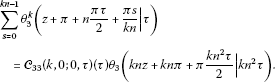(3.32)

Substituting n by $2n+1$ in the left-hand side of (3.32), we get (3.31). □

## References

1. Chan HH, Liu ZG, Ng ST: Circular summation of theta functions in Ramanujan’s lost notebook. J. Math. Anal. Appl. 2006, 316: 628-641. 10.1016/j.jmaa.2005.05.015

2. Zeng XF: A generalized circular summation of theta function and its application. J. Math. Anal. Appl. 2009, 356: 698-703. 10.1016/j.jmaa.2009.03.047

3. Liu ZG: Some inverse relations and theta function identities. Int. J. Number Theory 2012, 8: 1977-2002. 10.1142/S1793042112501126

4. Berndt BC: Ramanujan’s Notebooks, Part III. Springer, New York; 1991.

5. Berndt BC: Ramanujan’s Notebooks, Part V. Springer, New York; 1998.

6. Chan SH, Liu ZG: On a new circular summation of theta functions. J. Number Theory 2010, 130: 1190-1196. 10.1016/j.jnt.2009.09.011

7. Chua KS:The root lattice ${A}_{n}^{\ast }$ and Ramanujan’s circular summation of theta functions. Proc. Am. Math. Soc. 2002, 130: 1-8. 10.1090/S0002-9939-01-06080-4

8. Chua KS: Circular summation of the 13th powers of Ramanujan’s theta function. Ramanujan J. 2001, 5: 353-354. 10.1023/A:1013935519780

9. Liu ZG: A theta function identity and its implications. Trans. Am. Math. Soc. 2005, 357: 825-835. 10.1090/S0002-9947-04-03572-X

10. Ono K: On the circular summation of the eleventh powers of Ramanujan’s theta function. J. Number Theory 1999, 76: 62-65. 10.1006/jnth.1998.2354

11. Ramanujan S: The Lost Notebook and Other Unpublished Papers. Narosa, New Delhi; 1988.

12. Shen LC: On the additive formula of the theta functions and a collection of Lambert series pertaining to the modular equations of degree 5. Trans. Am. Math. Soc. 1994, 345: 323-345.

13. Son SH: Circular summation of theta functions in Ramanujan’s lost notebook. Ramanujan J. 2004, 8: 235-272.

14. Zhu JM: An alternate circular summation formula of theta functions and its applications. Appl. Anal. Discrete Math. 2012, 6: 114-125. 10.2298/AADM120204004Z

15. Zhu JM: A note on a generalized circular summation formula of theta functions. J. Number Theory 2012, 132: 1164-1169. 10.1016/j.jnt.2011.12.016

## Acknowledgements

Dedicated to Professor Hari M Srivastava.

The present investigation was supported, in part, by Research Project of Science and Technology of Chongqing Education Commission, China under Grant KJ120625, Fund of Chongqing Normal University, China under Grant 10XLR017 and 2011XLZ07 and National Natural Science Foundation of China under Grant 11226281 and 11271057.

## Author information

Authors

### Corresponding author

Correspondence to Qiu-Ming Luo.

### Competing interests

The authors declare that they have no competing interests.

### Authors’ contributions

All authors contributed equally in writing this paper, and read and approved the final manuscript and.

## Rights and permissions

Reprints and Permissions

Cai, Y., Chen, S. & Luo, QM. Some circular summation formulas for theta functions. Bound Value Probl 2013, 59 (2013). https://doi.org/10.1186/1687-2770-2013-59

• Accepted:

• Published:

• DOI: https://doi.org/10.1186/1687-2770-2013-59

### Keywords

• circular summation
• elliptic functions
• theta functions
• theta function identities